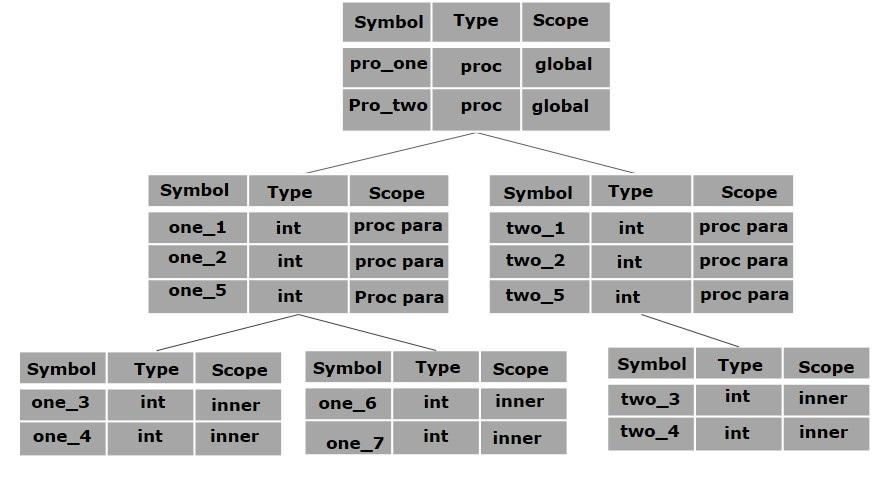# Compiler Design - Symbol Table

Symbol table is an important data structure created and maintained by compilers in order to store information about the occurrence of various entities such as variable names, function names, objects, classes, interfaces, etc. Symbol table is used by both the analysis and the synthesis parts of a compiler.

A symbol table may serve the following purposes depending upon the language in hand:

• To store the names of all entities in a structured form at one place.

• To verify if a variable has been declared.

• To implement type checking, by verifying assignments and expressions in the source code are semantically correct.

• To determine the scope of a name (scope resolution).

A symbol table is simply a table which can be either linear or a hash table. It maintains an entry for each name in the following format:

```<symbol name,  type,  attribute>
```

For example, if a symbol table has to store information about the following variable declaration:

```static int interest;
```

then it should store the entry such as:

```<interest, int, static>
```

The attribute clause contains the entries related to the name.

## Implementation

If a compiler is to handle a small amount of data, then the symbol table can be implemented as an unordered list, which is easy to code, but it is only suitable for small tables only. A symbol table can be implemented in one of the following ways:

• Linear (sorted or unsorted) list
• Binary Search Tree
• Hash table

Among all, symbol tables are mostly implemented as hash tables, where the source code symbol itself is treated as a key for the hash function and the return value is the information about the symbol.

## Operations

A symbol table, either linear or hash, should provide the following operations.

### insert()

This operation is more frequently used by analysis phase, i.e., the first half of the compiler where tokens are identified and names are stored in the table. This operation is used to add information in the symbol table about unique names occurring in the source code. The format or structure in which the names are stored depends upon the compiler in hand.

An attribute for a symbol in the source code is the information associated with that symbol. This information contains the value, state, scope, and type about the symbol. The insert() function takes the symbol and its attributes as arguments and stores the information in the symbol table.

For example:

```int a;
```

should be processed by the compiler as:

```insert(a, int);
```

### lookup()

lookup() operation is used to search a name in the symbol table to determine:

• if the symbol exists in the table.
• if it is declared before it is being used.
• if the name is used in the scope.
• if the symbol is initialized.
• if the symbol declared multiple times.

The format of lookup() function varies according to the programming language. The basic format should match the following:

```lookup(symbol)
```

This method returns 0 (zero) if the symbol does not exist in the symbol table. If the symbol exists in the symbol table, it returns its attributes stored in the table.

## Scope Management

A compiler maintains two types of symbol tables: a global symbol table which can be accessed by all the procedures and scope symbol tables that are created for each scope in the program.

To determine the scope of a name, symbol tables are arranged in hierarchical structure as shown in the example below:

```. . .
int value=10;

void pro_one()
{
int one_1;
int one_2;

{              \
int one_3;      |_  inner scope 1
int one_4;      |
}              /

int one_5;

{              \
int one_6;      |_  inner scope 2
int one_7;      |
}              /
}

void pro_two()
{
int two_1;
int two_2;

{              \
int two_3;      |_  inner scope 3
int two_4;      |
}              /

int two_5;
}
. . .
```

The above program can be represented in a hierarchical structure of symbol tables:The global symbol table contains names for one global variable (int value) and two procedure names, which should be available to all the child nodes shown above. The names mentioned in the pro_one symbol table (and all its child tables) are not available for pro_two symbols and its child tables.

This symbol table data structure hierarchy is stored in the semantic analyzer and whenever a name needs to be searched in a symbol table, it is searched using the following algorithm:

• first a symbol will be searched in the current scope, i.e. current symbol table.

• if a name is found, then search is completed, else it will be searched in the parent symbol table until,

• either the name is found or global symbol table has been searched for the name.